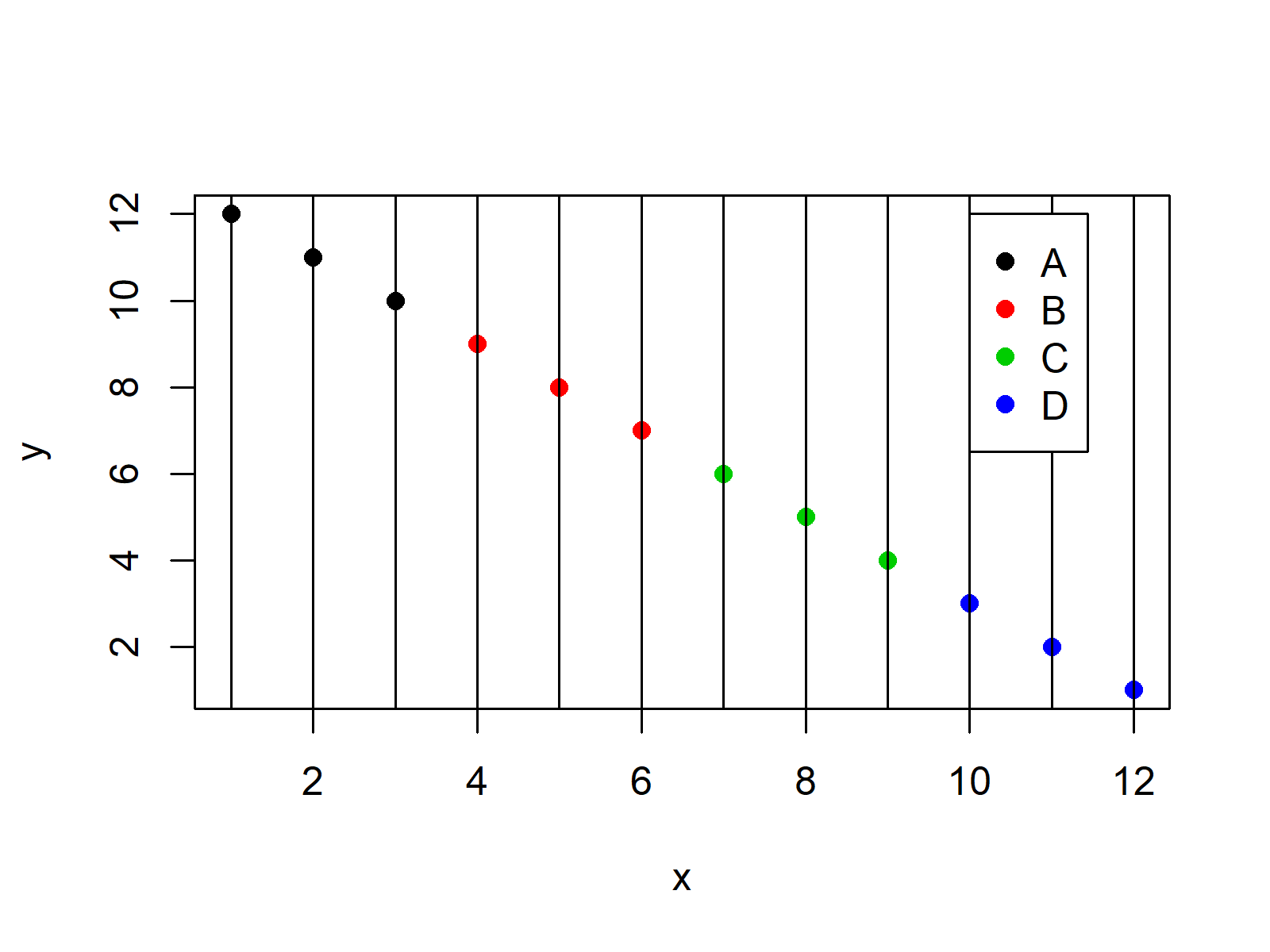# Add Legend without Border & White Background to Plot in R (Example)

In this tutorial, I’ll explain how to add a legend with white border and background to a graph in R.

The page contains the following content blocks:

So let’s do this.

## Example Data

We use the following data as basement for this R tutorial:

```x <- 1:12                        # Create example data
y <- 12:1
group <- rep(1:4, each = 3)```

Now, we can draw our data in a Base R scatterplot as follows:

```plot(x, y,                       # Draw data
col = group,
pch = 16)
abline(v = 1:12)                 # Adding ablines to plot
legend(x = 10,                   # Add legend with borders
y = 12,
legend = LETTERS[1:4],
col = 1:4,
pch = 16)```Figure 1 visualizes the output of the previous code – A plot that contains a default legend with borders.

## Example: Adding Legend without Borders & White Background to Plot

This example explains how to draw a legend without borders and a white background to our graphic. Have a look at the following R code and the resulting graph:

```plot(x, y,                       # Draw data
col = group,
pch = 16)
abline(v = 1:12)                 # Adding ablines to plot
legend(x = 10,                   # Add legend without borders
y = 12,
legend = LETTERS[1:4],
col = 1:4,
pch = 16,
box.col = "white")        # White border color```As revealed in Figure 2, the previous R code created a legend with white border and background. All we had to do was to add the box.col = “white” argument to the legend function.

## Video, Further Resources & Summary

Have a look at the following video of my YouTube channel. In the video tutorial, I’m illustrating the R programming codes of this tutorial:

Please accept YouTube cookies to play this video. By accepting you will be accessing content from YouTube, a service provided by an external third party.If you accept this notice, your choice will be saved and the page will refresh.

Additionally, you may have a look at the other tutorials of this website:

To summarize: On this page you learned how to draw a white legend in R programming. Let me know in the comments section, if you have any additional questions.

Subscribe to the Statistics Globe Newsletter